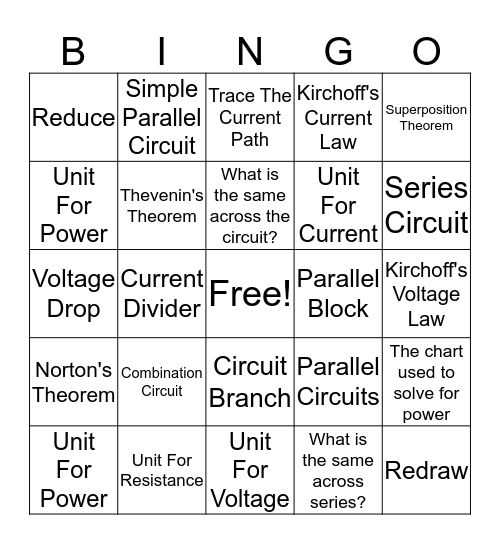# Unit 8 + 9 BingoThis bingo card has a free space and 25 words: Combination Circuit, Node, Parallel Block, Redraw, Reduce, Simple Parallel Circuit, Trace The Current Path, Kirchoff's Current Law, Kirchoff's Voltage Law, Norton's Theorem, Thevenin's Theorem, What is the same across the circuit?, What is the same across series?, The chart used to solve for power, Unit For Power, Unit For Resistance, Unit For Voltage, Unit For Current, Series Circuit, Voltage Drop, Current Divider, Circuit Branch, Parallel Circuits, Superposition Theorem and Unit For Power.

⚠ This card has duplicate items: Unit For Power (2)

## Play Online